## Exercises

1. Let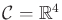. There are two Pfaffian constraints,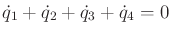and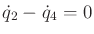. Determine the appropriate number of action variables and express the differential constraints in the form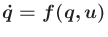.
2. Introduce a phase space and convert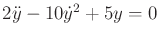into the form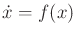.
3. Introduce a phase space and convert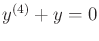into the form.
4. Derive the configuration transition equation (13.19) for a car pulling trailers.
5. Use the main idea of Section 13.2.4 to develop a smooth-steering extension of the car pulling trailers, (13.19).
6. Suppose that two identical differential-drive robots are connected together at their centers with a rigid bar of length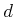. The robots are attached at each end of the rod, and each attachment forms a revolute joint. There are four wheels to control; however, some combinations of wheel rotations cause skidding. Assuming that skidding is not allowed, develop a motion model of the form, in whichand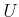are chosen to reflect the true degrees of freedom.
7. Extend the lunar lander model to a general rigid body with a thruster that does not apply forces through the center of mass.
8. Develop a model for a 3D rotating rigid body fired out of a canon at a specified angle above level ground under gravity. Suppose that thrusters are placed on the body, enabling it to be controlled before it impacts the ground. Develop general phase transition equations.
9. Add gravity with respect to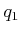in Example 13.12 and derive the new state transition equation using the Euler-Lagrange equation.
10. Use the constrained Lagrangian to derive the equations of motion of the pendulum in Example 13.8.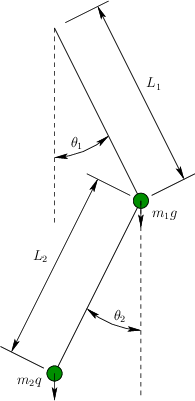11. Define a phase space, and determine an equation of the formfor the double pendulum shown in Figure 13.14.
12. Extend Example 13.13 to obtain the dynamics of a three-link manipulator. The third link,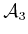, is attached to the other two by a revolute joint. The new parameters are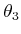,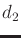,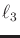,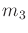, and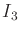.
13. Solve Example 13.14 by parameterizing the sphere with standard spherical coordinates and using the unconstrained Lagrangian. Verify that the same answer is obtained.
14. Convert the equations in (13.161) into phase space form, to obtain the phase transition equation in the form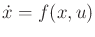. Express the right side of the equation in terms of the basic parameters, such as mass, moment of inertia, and lengths.
15. Define the Hamiltonian for a free-floating 2D rigid body under gravity and develop Hamilton's equations.

Implementations

16. Make a 3D spacecraft (rigid-body) simulator that allows any number of binary thrusters to be placed in any position and orientation.
17. Make a simulator for the two-link manipulator in Example 13.13.

Steven M LaValle 2020-08-14